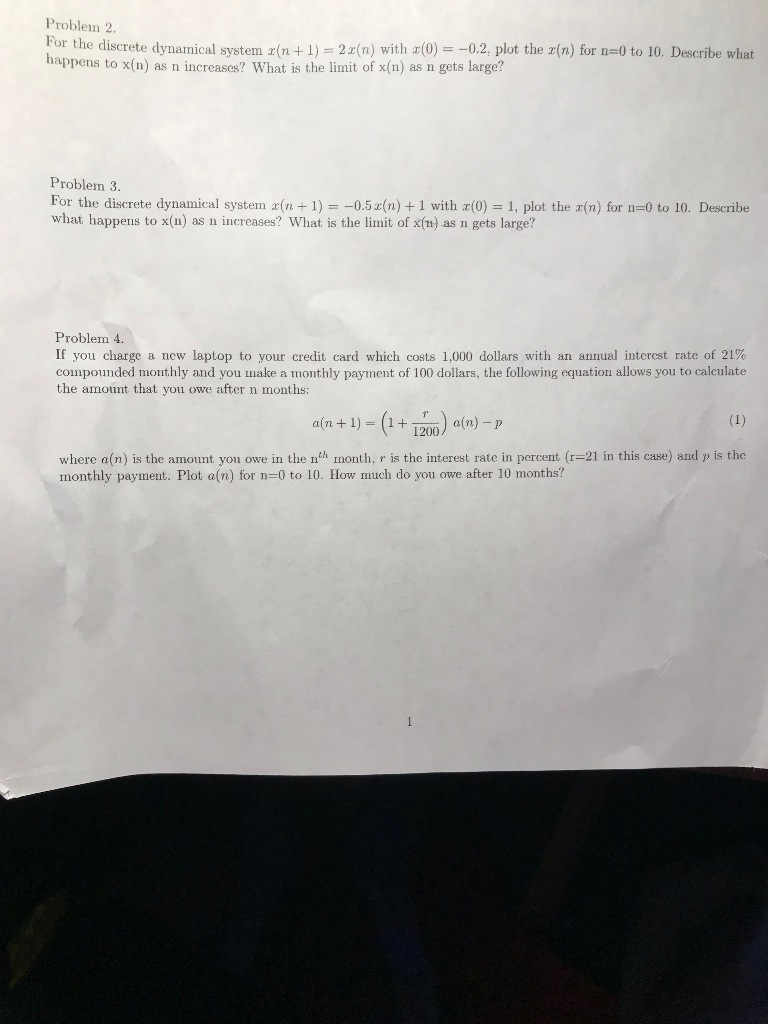Homework Help Question & Answers

Only Problem 2!!! MATLAB only and please comment as well. Received this problem for homework and ...Only Problem 2!!! MATLAB only and please comment as well. Received this problem for homework and am struggling!

Problem 2. or the discrete dynamical system (n+1)2(n) with a(0)0.2, plot the z(n) for n-0 to 10. Describe what happens to x(n) as n increases? What is the limit of x(n) as n gets large? Problem 3. For the discrete dynamical system n + 1)5+1 with (0) 1, plot the r(n) for n-0 to 10. Describe what happens to x(n) as n increases? What is the limit of x(n) as n gets large? Problem 4 If you charge a new laptop to your credit card which costs 1.000 dollars with an annual interest rate of 21% compounded monthly and you make a monthly payment of 100 dollars, the following equation allows you to calculate the amount that you owe after n months: a(n + 1)-(1 + 1200) a(n)-p where a(n) is the amount you owe in the nth month, r is the interest rate in percent (r-21 in this case) and p is the monthly payment. Plot a(n) for n-0 to 10. How m uch do you owe after 10 months?

%%%%matlab code

cla;

clear all;

close all;

x(1)=-0.2

For n= 1:9

x(n+1)= 2*x(n);

end

Figure ;

stem(0:9,x);

xlabel('n')

ylabel('x')

As n increase, value of x(n) also increase but in negative

Direction ...

D

Add Answer of: Only Problem 2!!! MATLAB only and please comment as well. Received this problem for homework and ...
More Homework Help Questions Additional questions in this topic.

• MATLAB ONLY MATLAB ONLY 2. (15 pts) Let b (1) n! 20 1) (5pts) Compute b and b 2) (5pts)Let Sb and S-b find the least N in natural numer s.t k-1 S -S 0.01 3) (5pts) Plot the constant function which...

Need Online Homework Help?

Get FREE EXPERT Answers
WITHIN MINUTES
Related Questions